# G.4 - 6 Which Trig Ratio?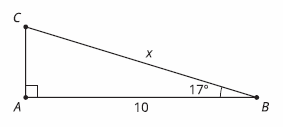Which ratio could be used to write an equation relating the sides and angle given in this picture?

Select all that apply
• A
• B
• C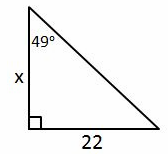Which ratio could be used to write an equation relating the sides and angle given in this picture?

Select all that apply
• A
• B
• C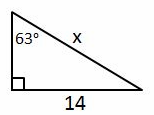Which ratio could be used to write an equation relating the sides and angle given in this picture?

Select all that apply
• A
• B
• C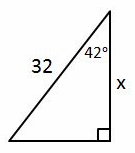Which ratio could be used to write an equation relating the sides and angle given in this picture?

Select all that apply
• A
• B
• C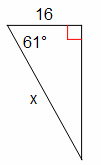Which ratio could be used to write an equation relating the sides and angle given in this picture?

Select all that apply
• A
• B
• C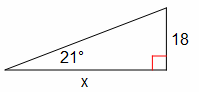Which ratio could be used to write an equation relating the sides and angle given in this picture?

Select all that apply
• A
• B
• C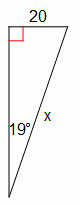Which ratio could be used to write an equation relating the sides and angle given in this picture?

Select all that apply
• A
• B
• C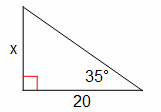Which ratio could be used to write an equation relating the sides and angle given in this picture?

Select all that apply
• A
• B
• C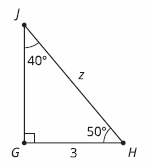Which two ratios could be used to write an equation relating 2 sides and an angle given in this picture?

Select all that apply
• A
• B
• C